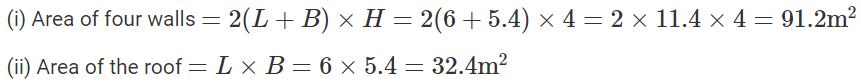# Selina Solutions Concise Maths Class 8 Chapter 21: Surface Area, Volume and Capacity

Selina Solutions Concise Maths Class 8 Chapter 21 Surface Area, Volume and Capacity consists of accurate answers to the problems present in the textbook. Each and every problem is solved with utmost care with the aim of helping students to ace their exams without fear. To get a better hold on the concepts covered in this chapter, students can access the ICSE Selina Solutions Class 8 Maths Chapter 21 Surface Area, Volume and Capacity free PDF, from the link which is given below.

This chapter has the concepts explained in a comprehensive manner to make learning fun for the students. It is highly recommended for the Class 8 students to solve the questions present in the textbook using the solutions for a better understanding of the concepts. It will also help the students with their analytical and logical thinking abilities, which is necessary to perform well in the annual exam.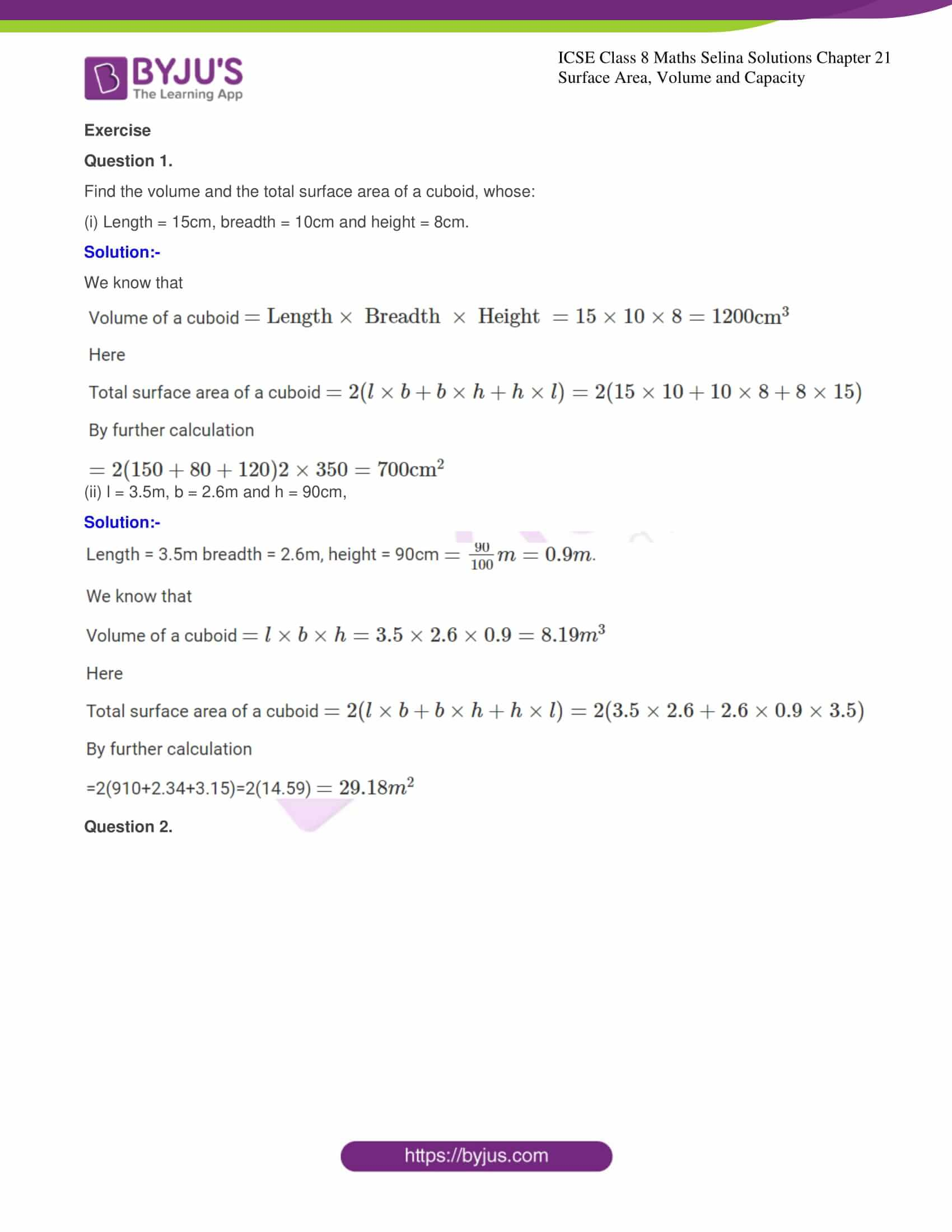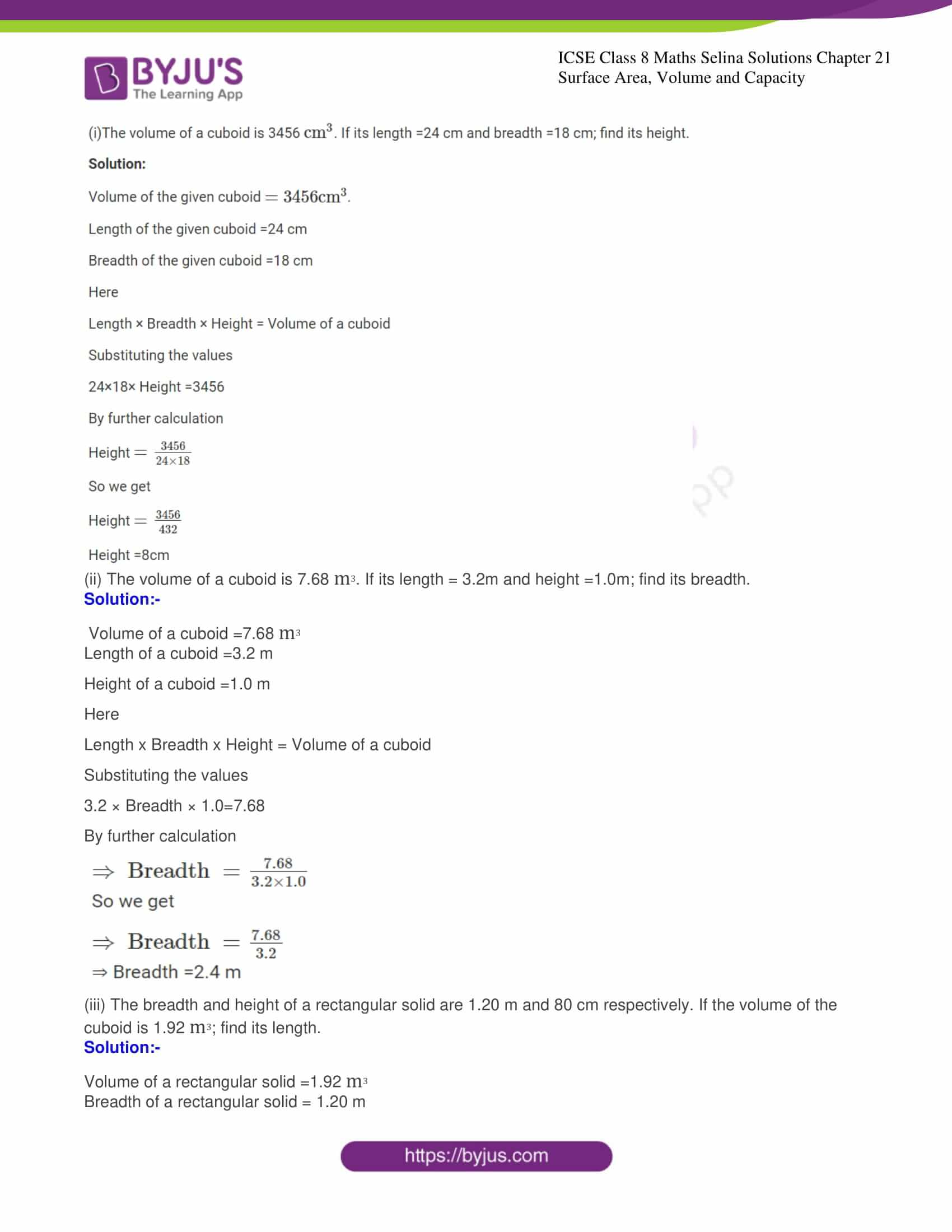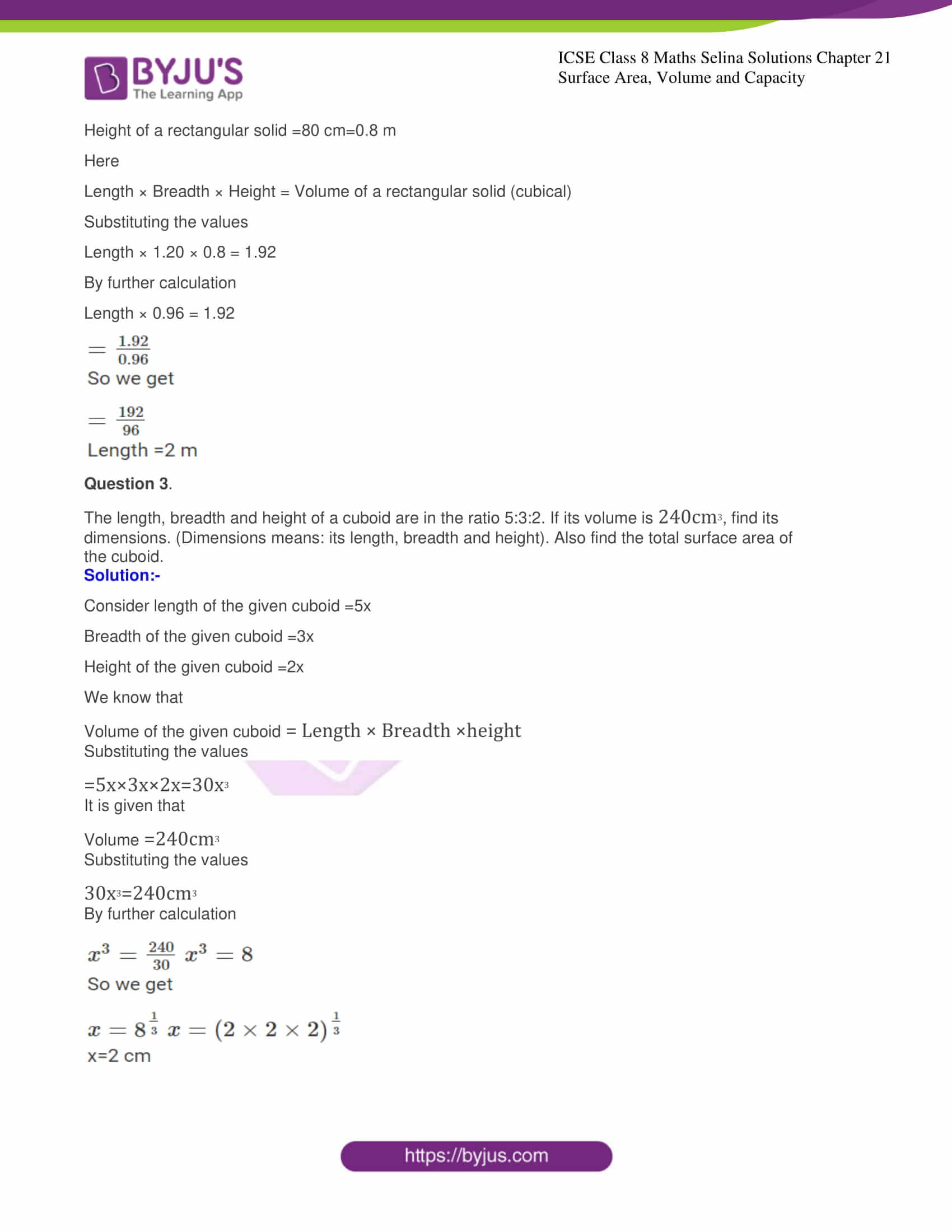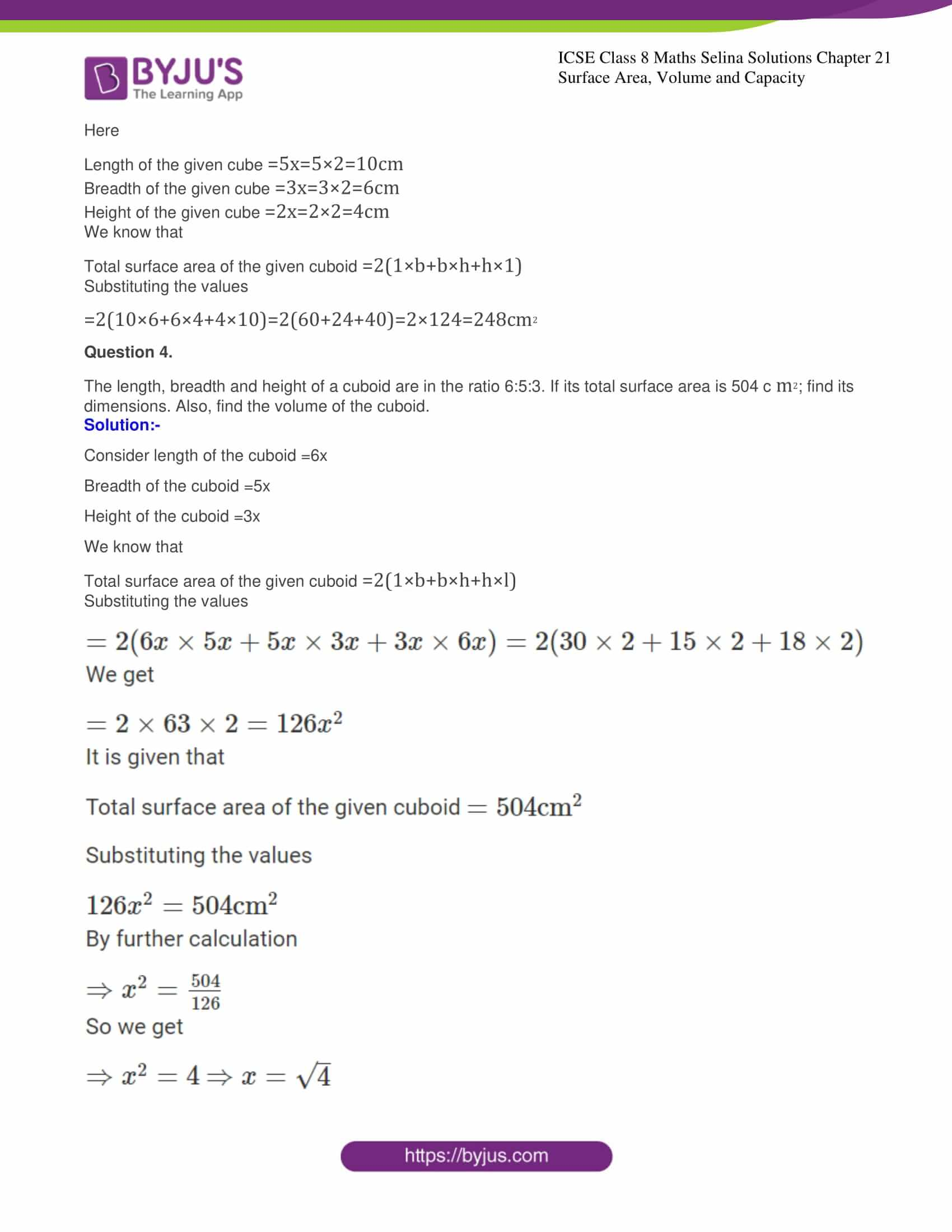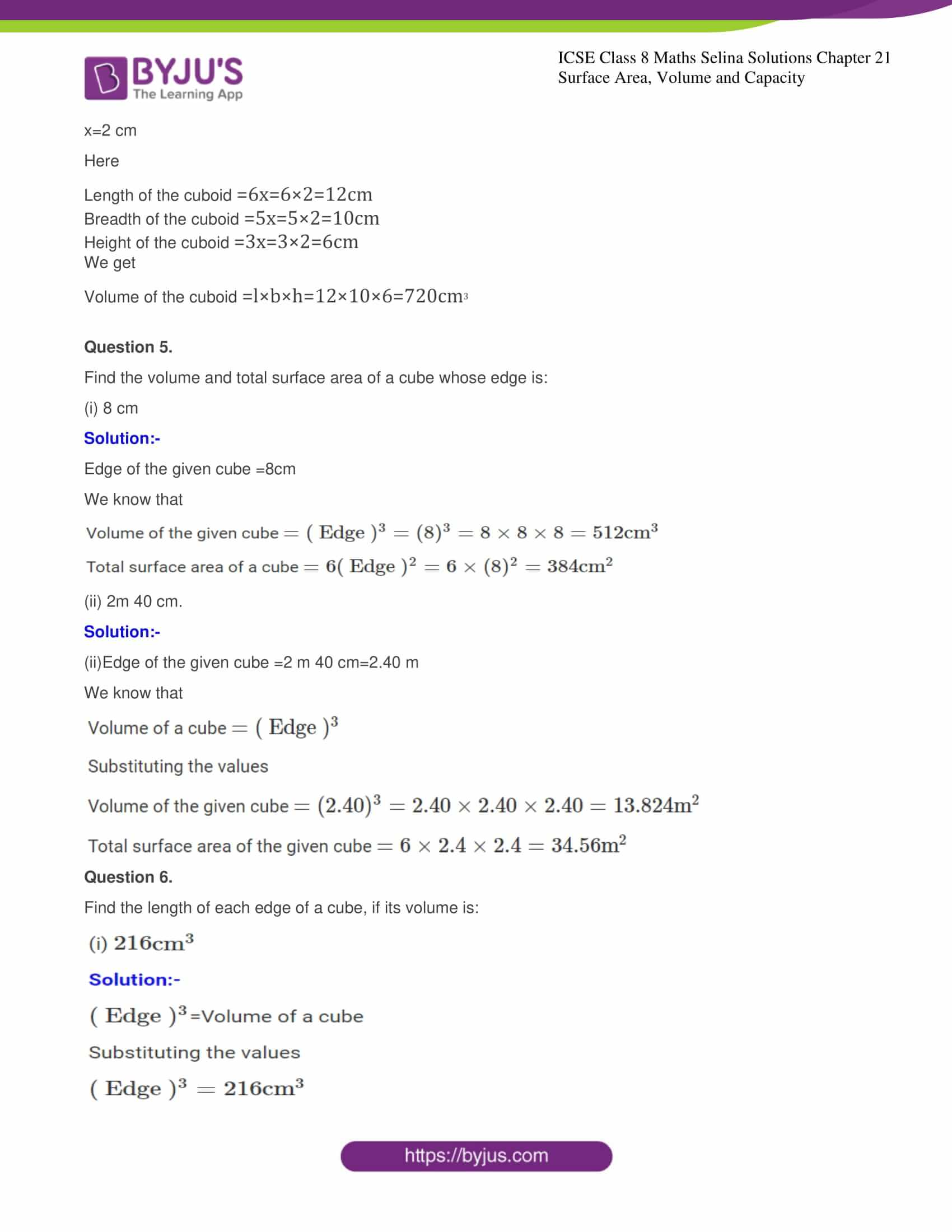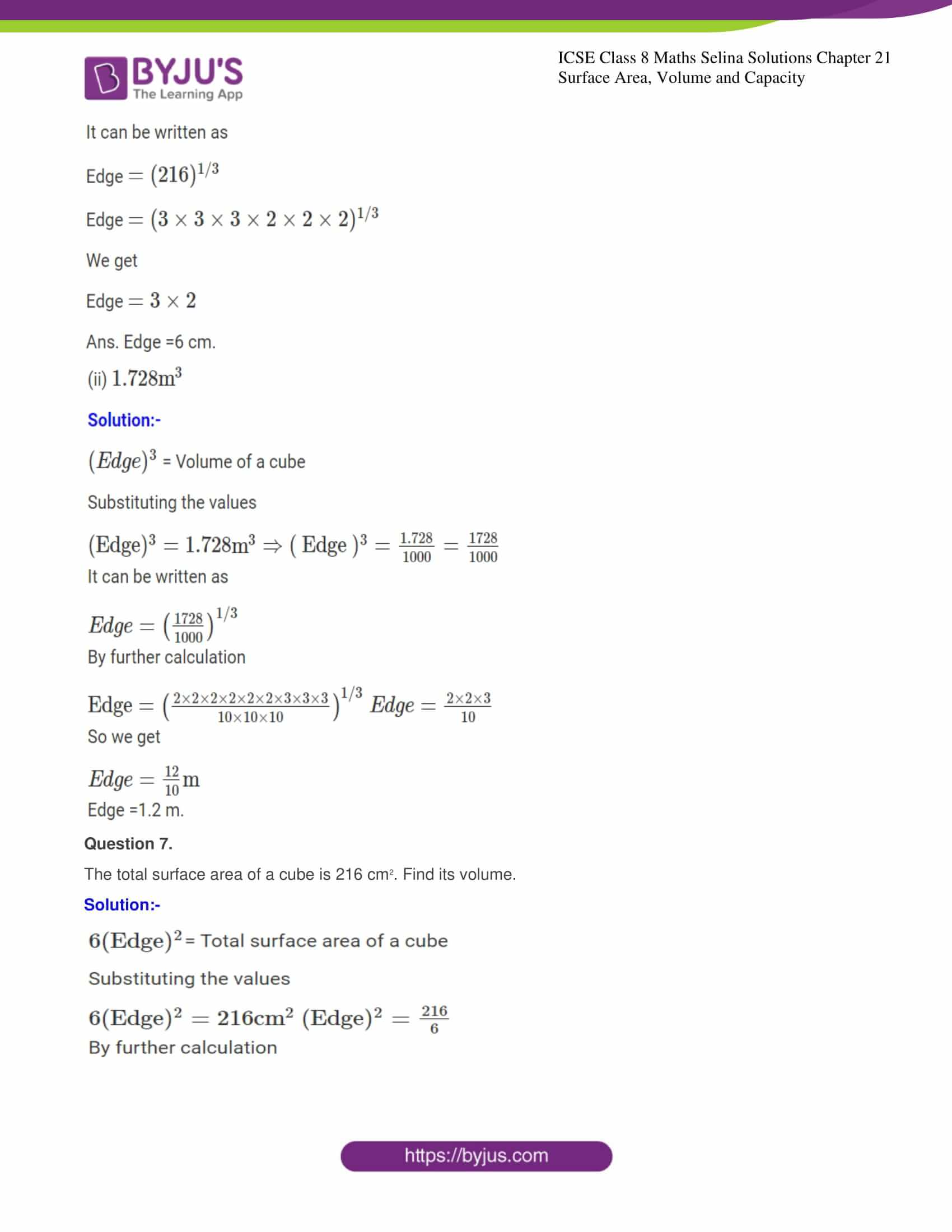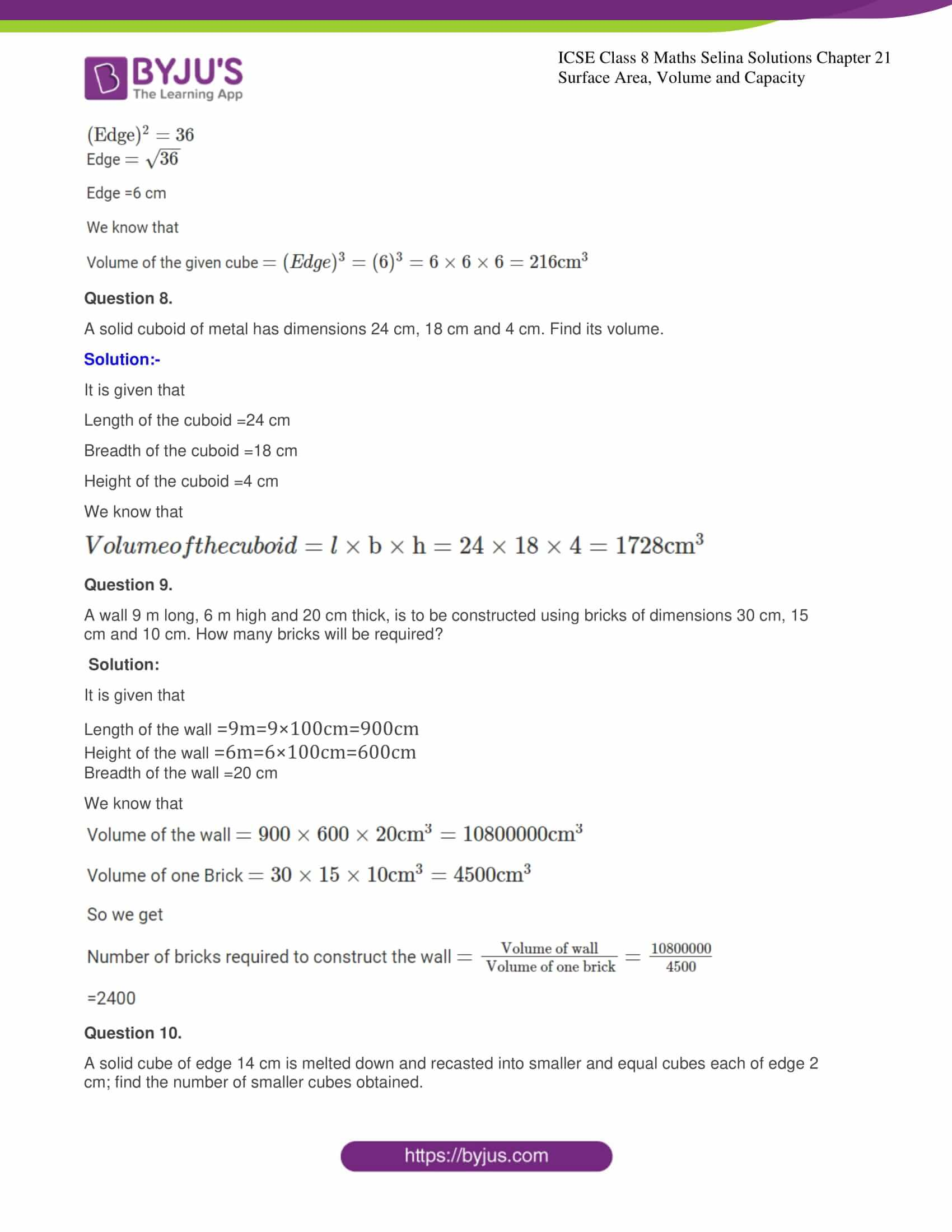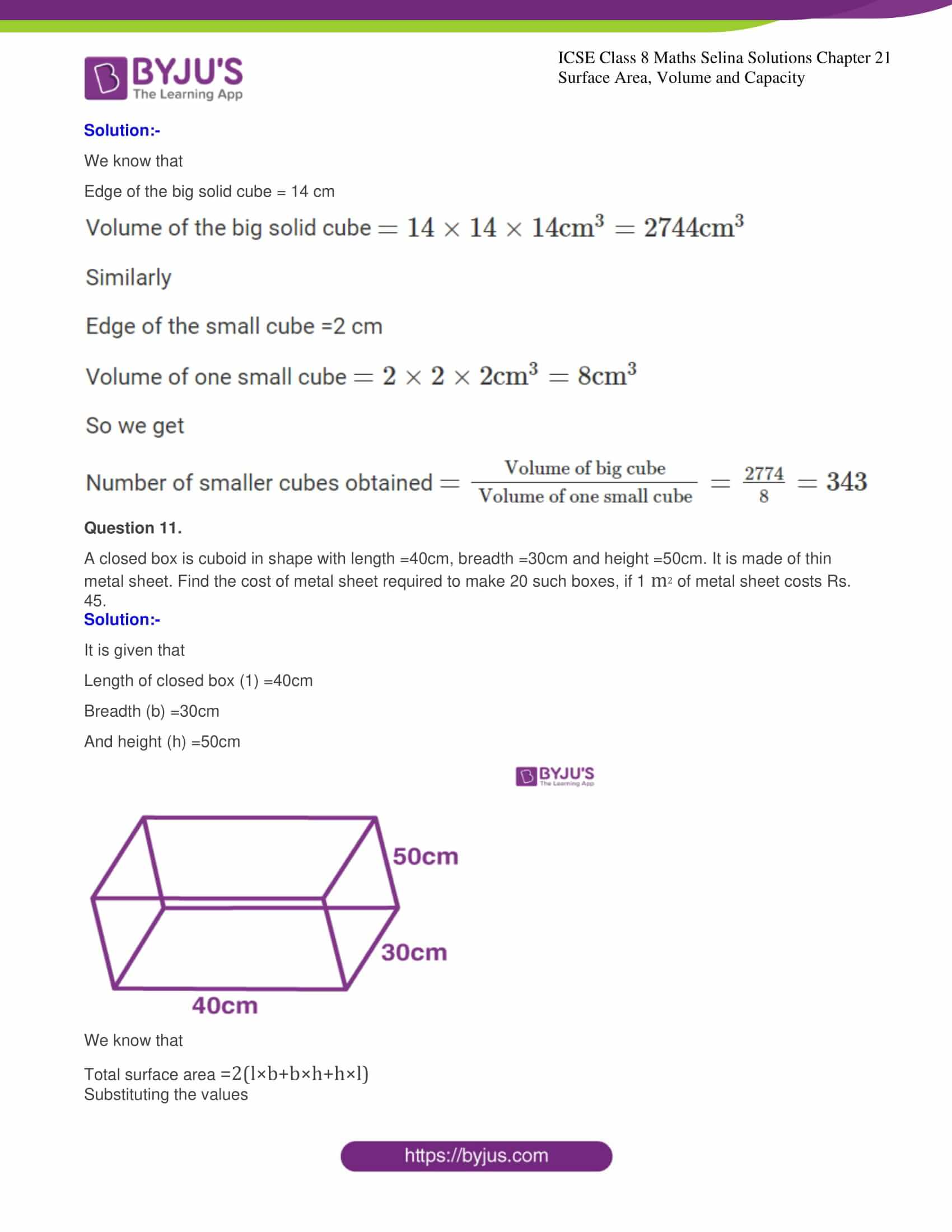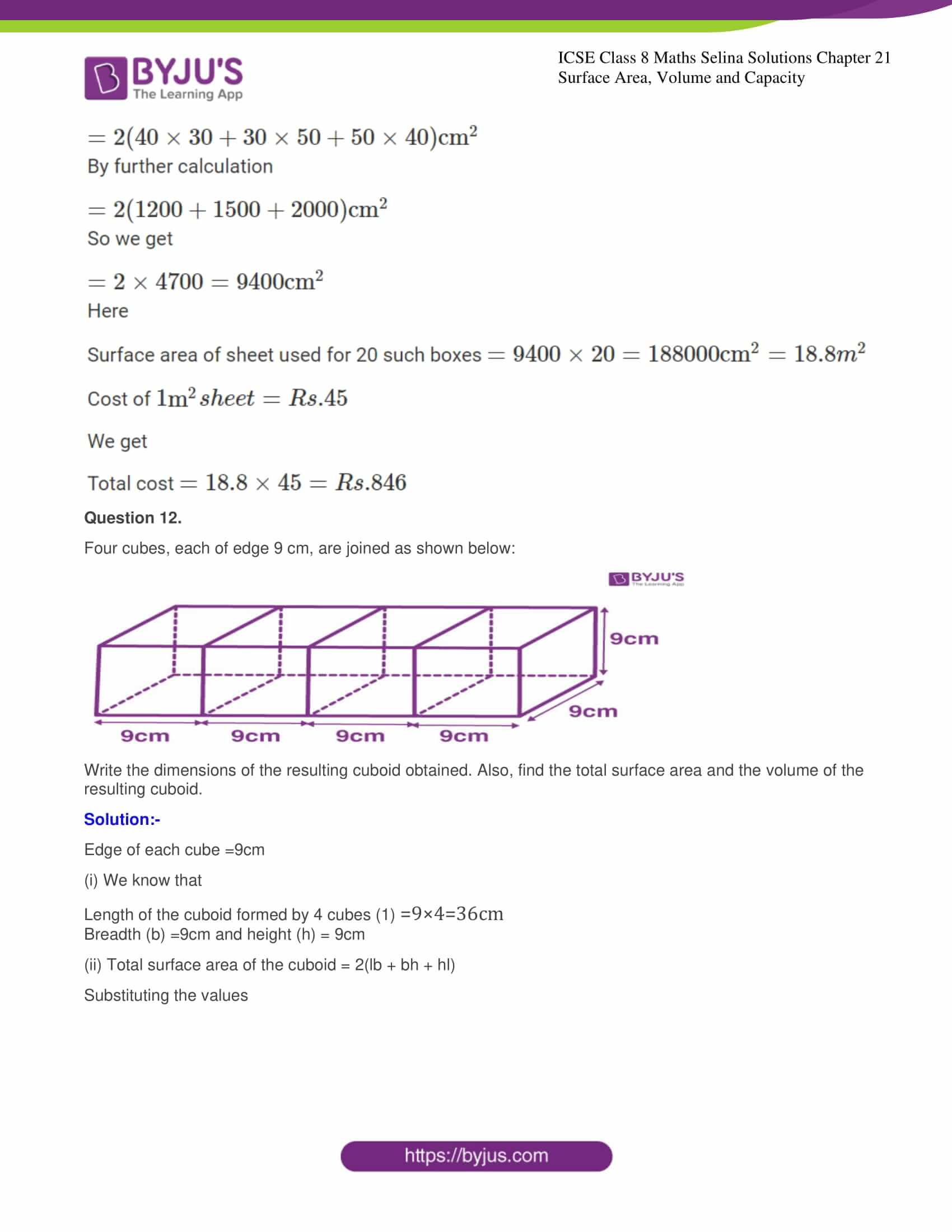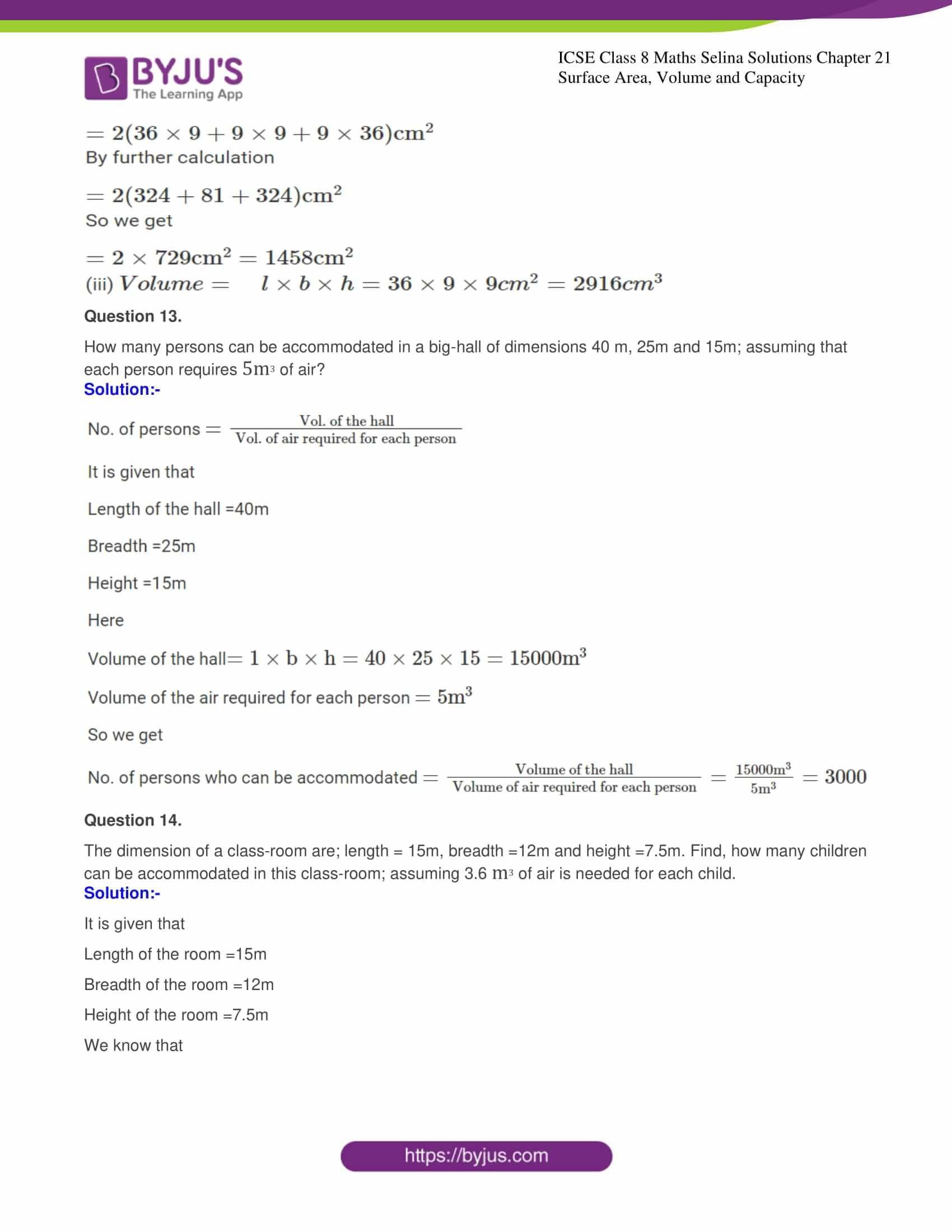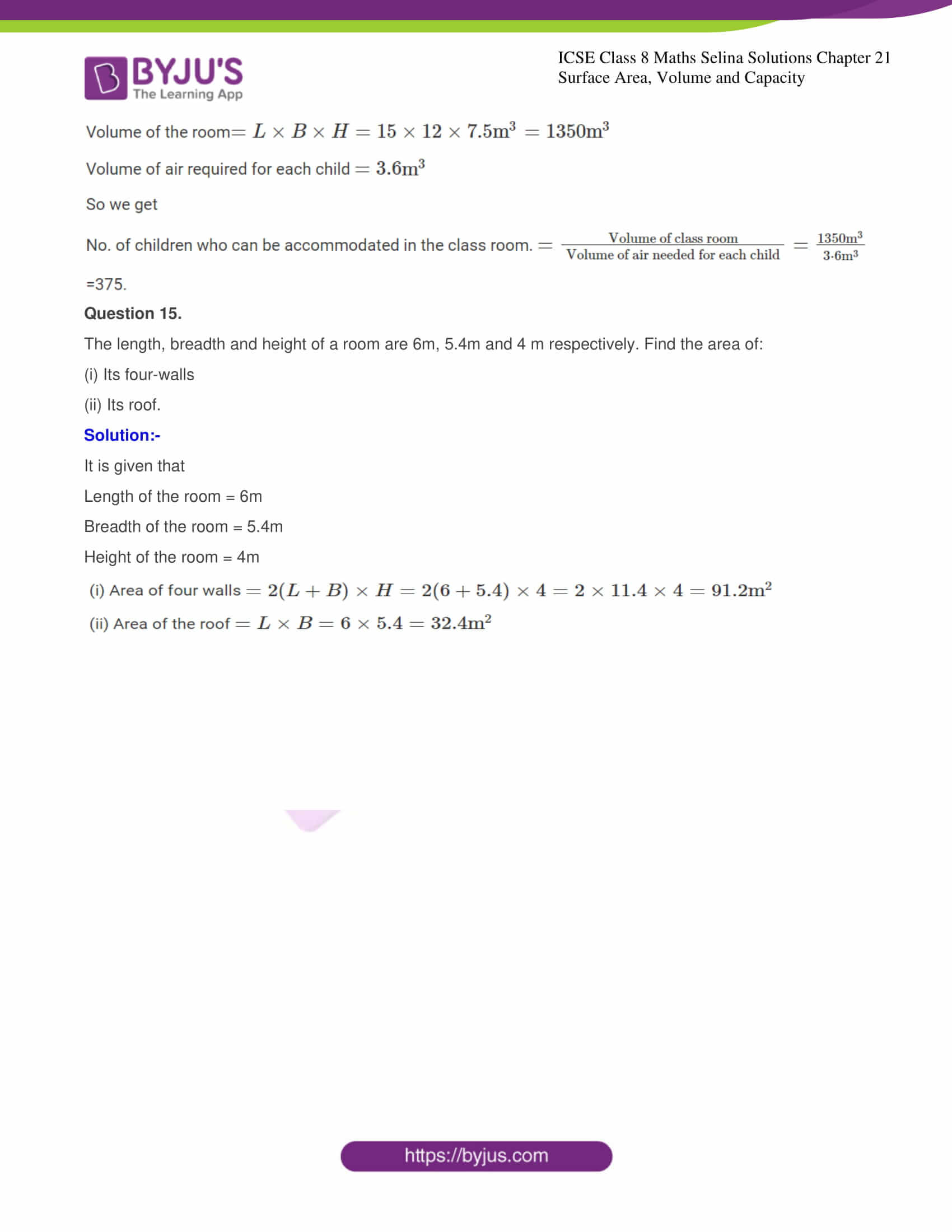## Access Selina Solutions Concise Maths Class 8 Chapter 21: Surface Area, Volume and Capacity

Exercise

Question 1.

Find the volume and the total surface area of a cuboid, whose:

(i) Length = 15cm, breadth = 10cm and height = 8cm.

Solution:-

We know that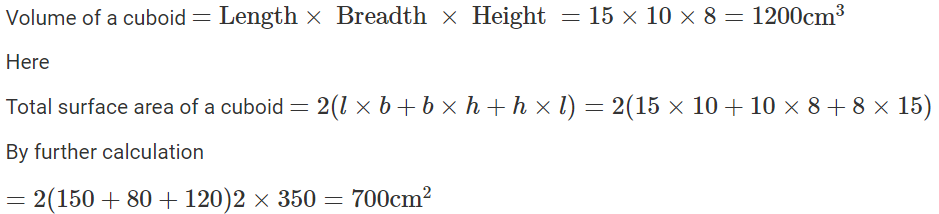(ii) l = 3.5m, b = 2.6m and h = 90cm,

Solution:-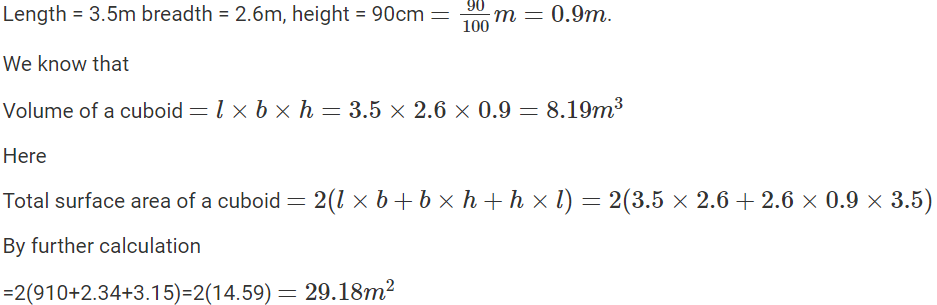Question 2.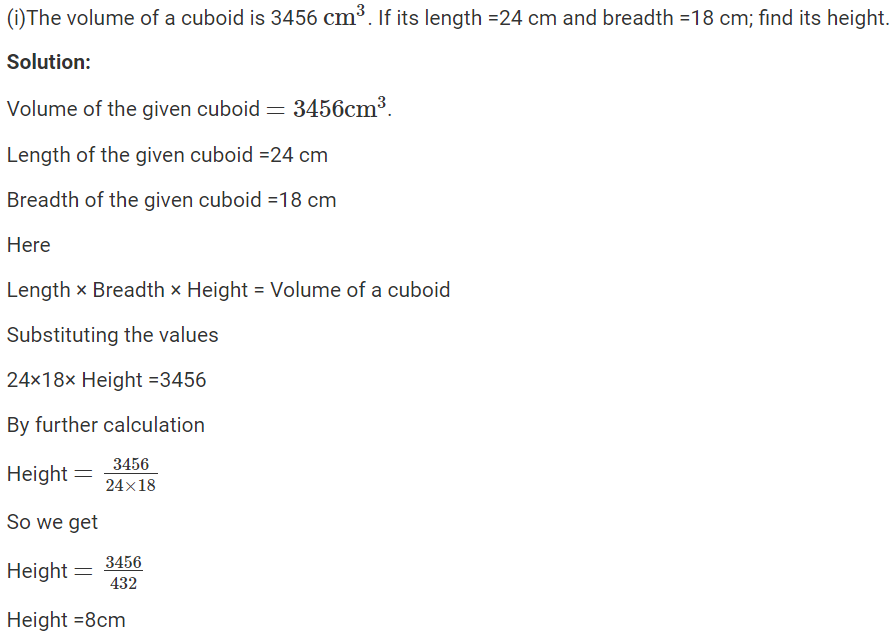(ii) The volume of a cuboid is 7.68 m3. If its length = 3.2m and height =1.0m; find its breadth.

Solution:-

Volume of a cuboid =7.68 m3

Length of a cuboid =3.2 m

Height of a cuboid =1.0 m

Here

Length x Breadth x Height = Volume of a cuboid

Substituting the values

By further calculation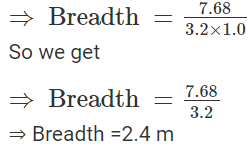(iii) The breadth and height of a rectangular solid are 1.20 m and 80 cm respectively. If the volume of the cuboid is 1.92 m3; find its length.

Solution:-

Volume of a rectangular solid =1.92 m3

Breadth of a rectangular solid = 1.20 m

Height of a rectangular solid =80 cm=0.8 m

Here

Length × Breadth × Height = Volume of a rectangular solid (cubical)

Substituting the values

Length × 1.20 × 0.8 = 1.92

By further calculation

Length × 0.96 = 1.92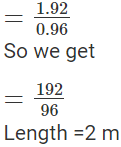Question 3.

The length, breadth and height of a cuboid are in the ratio 5:3:2. If its volume is 240cm3, find its dimensions. (Dimensions means: its length, breadth and height). Also find the total surface area of the cuboid.

Solution:-

Consider length of the given cuboid =5x

Breadth of the given cuboid =3x

Height of the given cuboid =2x

We know that

Volume of the given cuboid = Length × Breadth ×height

Substituting the values

=5x×3x×2x=30x3

It is given that

Volume =240cm3

Substituting the values

30x3=240cm3

By further calculation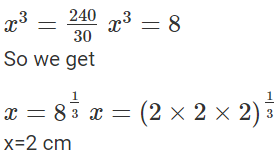Here

Length of the given cube =5x=5×2=10cm

Breadth of the given cube =3x=3×2=6cm

Height of the given cube =2x=2×2=4cm

We know that

Total surface area of the given cuboid =2(1×b+b×h+h×1)

Substituting the values

=2(10×6+6×4+4×10)=2(60+24+40)=2×124=248cm2

Question 4.

The length, breadth and height of a cuboid are in the ratio 6:5:3. If its total surface area is 504 c m2; find its dimensions. Also, find the volume of the cuboid.

Solution:-

Consider length of the cuboid =6x

Height of the cuboid =3x

We know that

Total surface area of the given cuboid =2(1×b+b×h+h×l)

Substituting the values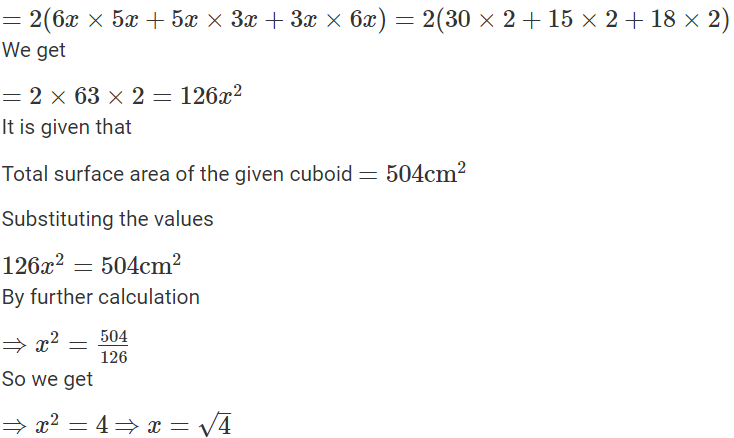x=2 cm

Here

Length of the cuboid =6x=6×2=12cm

Height of the cuboid =3x=3×2=6cm

We get

Volume of the cuboid =l×b×h=12×10×6=720cm3

Question 5.

Find the volume and total surface area of a cube whose edge is:

(i) 8 cm

Solution:-

Edge of the given cube =8cm

We know that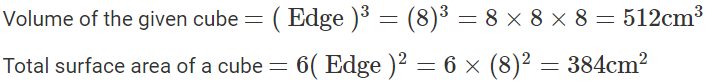(ii) 2m 40 cm.

Solution:-

(ii)Edge of the given cube =2 m 40 cm=2.40 m

We know that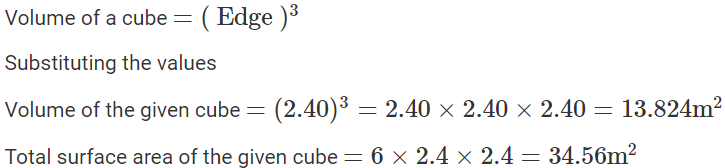Question 6.

Find the length of each edge of a cube, if its volume is: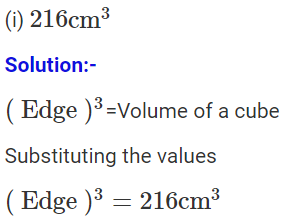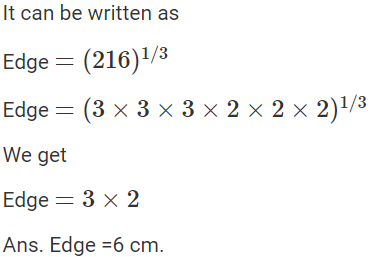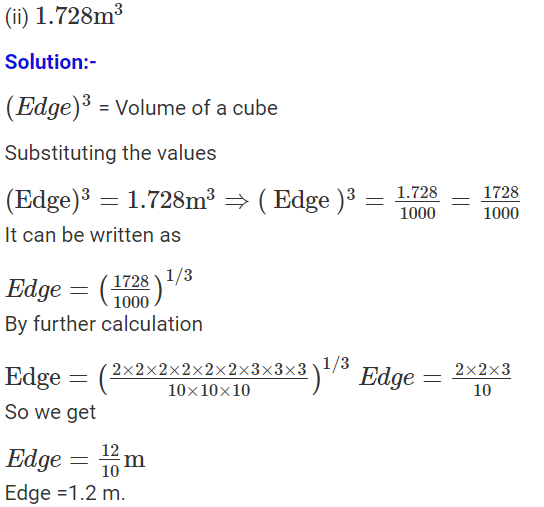Question 7.

The total surface area of a cube is 216 cm2. Find its volume.

Solution:-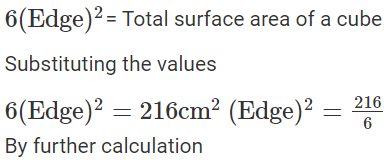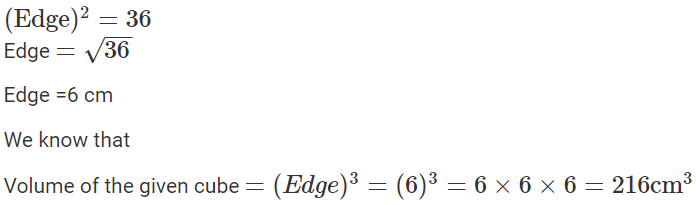Question 8.

A solid cuboid of metal has dimensions 24 cm, 18 cm and 4 cm. Find its volume.

Solution:-

It is given that

Length of the cuboid =24 cm

Breadth of the cuboid =18 cm

Height of the cuboid =4 cm

We know that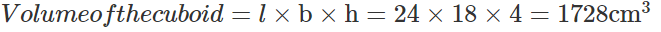Question 9.

A wall 9 m long, 6 m high and 20 cm thick, is to be constructed using bricks of dimensions 30 cm, 15 cm and 10 cm. How many bricks will be required?

Solution:

It is given that

Length of the wall =9m=9×100cm=900cm

Height of the wall =6m=6×100cm=600cm

Breadth of the wall =20 cm

We know that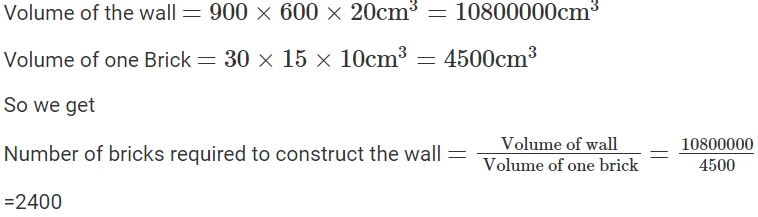Question 10.

A solid cube of edge 14 cm is melted down and recasted into smaller and equal cubes each of edge 2 cm; find the number of smaller cubes obtained.

Solution:-

We know that

Edge of the big solid cube = 14 cm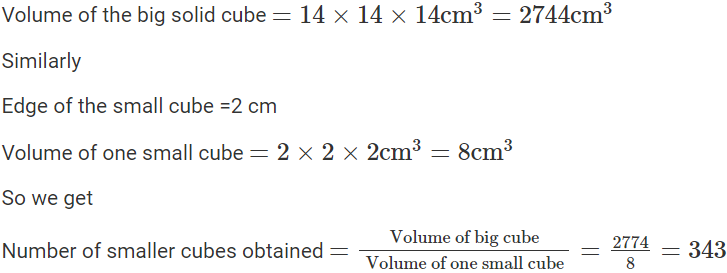Question 11.

A closed box is cuboid in shape with length =40cm, breadth =30cm and height =50cm. It is made of thin metal sheet. Find the cost of metal sheet required to make 20 such boxes, if 1 m2 of metal sheet costs Rs. 45.

Solution:-

It is given that

Length of closed box (1) =40cm

And height (h) =50cm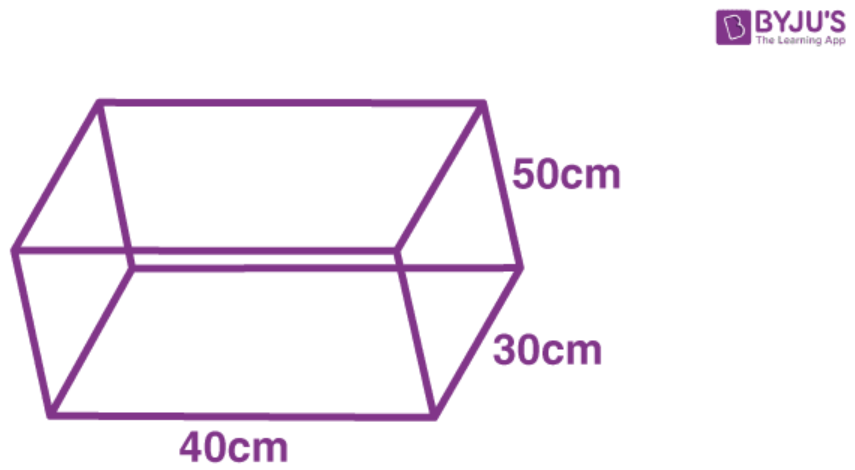We know that

Total surface area =2(l×b+b×h+h×l)

Substituting the values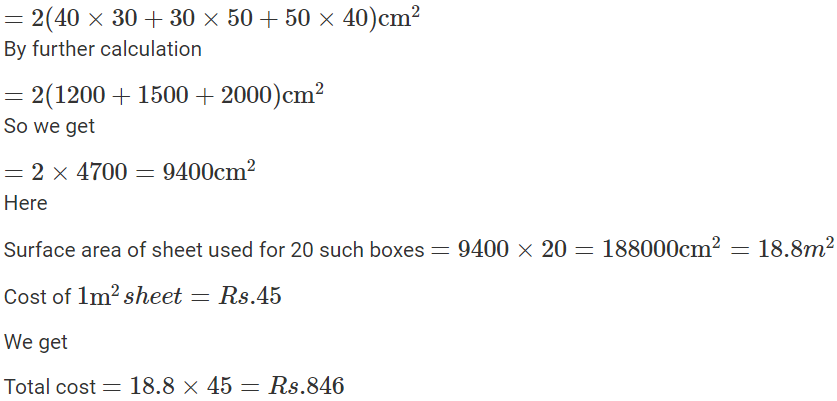Question 12.

Four cubes, each of edge 9 cm, are joined as shown below: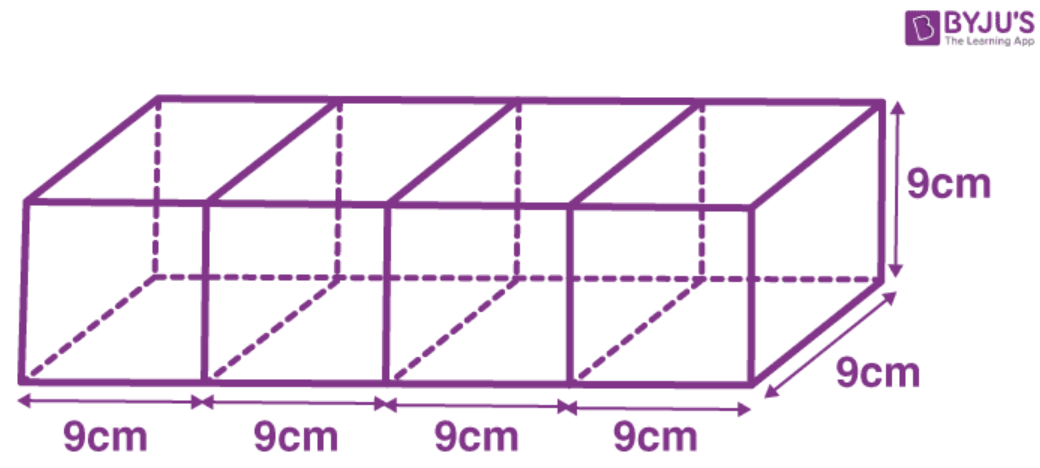Write the dimensions of the resulting cuboid obtained. Also, find the total surface area and the volume of the resulting cuboid.

Solution:-

Edge of each cube =9cm

(i) We know that

Length of the cuboid formed by 4 cubes (1) =9×4=36cm

Breadth (b) =9cm and height (h) = 9cm

(ii) Total surface area of the cuboid = 2(lb + bh + hl)

Substituting the values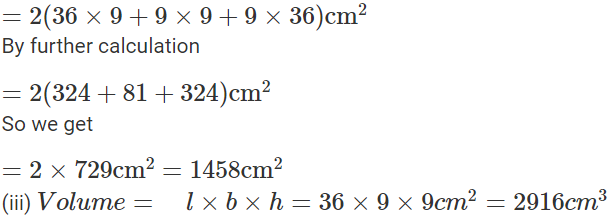Question 13.

How many persons can be accommodated in a big-hall of dimensions 40 m, 25m and 15m; assuming that each person requires 5m3 of air?

Solution:-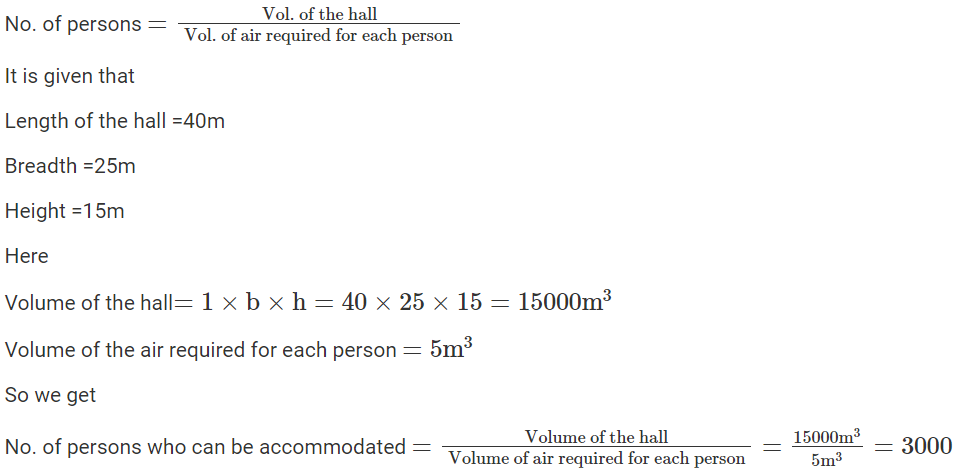Question 14.

The dimension of a class-room are; length = 15m, breadth =12m and height =7.5m. Find, how many children can be accommodated in this class-room; assuming 3.6 m3 of air is needed for each child.

Solution:-

It is given that

Length of the room =15m

Height of the room =7.5m

We know that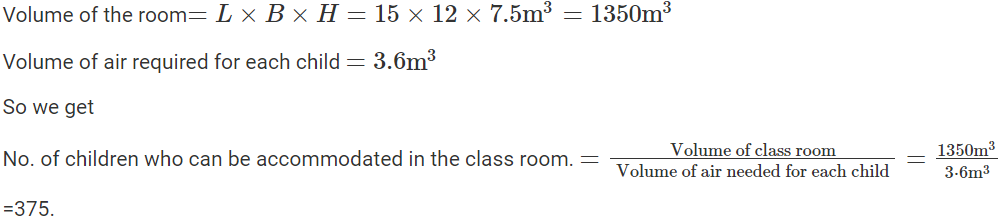Question 15.

The length, breadth and height of a room are 6m, 5.4m and 4 m respectively. Find the area of:

(i) Its four-walls

(ii) Its roof.

Solution:-

It is given that

Length of the room = 6m

Breadth of the room = 5.4m

Height of the room = 4m